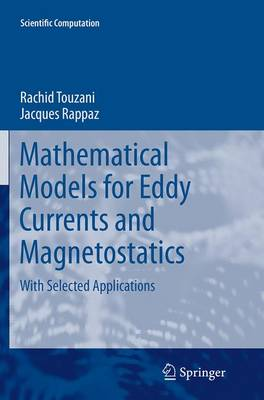•# Mathematical Models for Eddy Currents and Magnetostatics: With Selected Applications - Scientific Computation (Paperback)

(author), (author)
£72.00
Paperback 305 Pages / Published: 27/08/2016
• We can order this

Usually dispatched within 3 weeks

This monograph addresses fundamental aspects of mathematical modeling and numerical solution methods of electromagnetic problems involving low frequencies, i.e. magnetostatic and eddy current problems which are rarely presented in the applied mathematics literature. In the first part, the authors introduce the mathematical models in a realistic context in view of their use for industrial applications. Several geometric configurations of electric conductors leading to different mathematical models are carefully derived and analyzed, and numerical methods for the solution of the obtained problems are given. Related issues such as convergence of the approximations and error estimates are discussed. The second part of the monograph presents various coupled problems that involve eddy current or magnetostatic problems, in particular magneto-hydrodynamic problems and magnetic shaping problems concerning the melt flow of electrically conducting metals, induction heating processes, inductively coupled plasmas and ferromagnetic screening modeling. The presentation of each model comes with numerical illustration from industrial applications.

Publisher: Springer
ISBN: 9789401778169
Number of pages: 305
Weight: 4861 g
Dimensions: 235 x 155 x 17 mm
Edition: Softcover reprint of the original 1st ed. 201

MEDIA REVIEWS

From the book reviews:

"This textbook is devoted to the mathematical modeling and the efficient numerical solution of magnetostatic and eddy current problems. ... The book is directed to applied mathematicians, and to researchers and developers in electric and electrotechnical engineering as well." (Johannes Elschner, zbMATH, Vol. 1288, 2014)